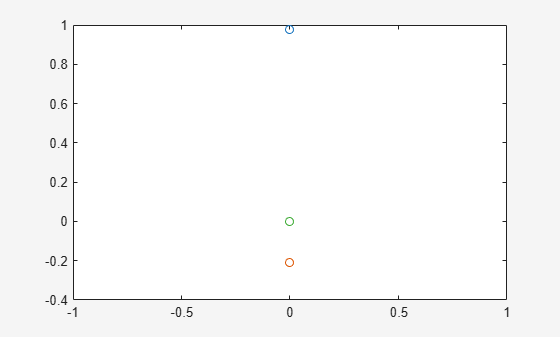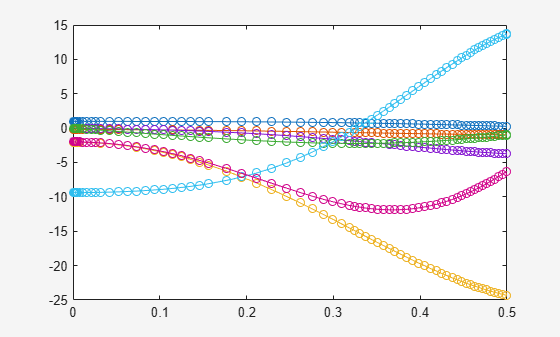# Analyze and Manipulate Differential Algebraic Equations

This example shows how to solve differential algebraic equations (DAEs) of high differential index using Symbolic Math Toolbox™.

Engineers often specify the behavior of their physical objects (mechanical systems, electrical devices, and so on) by a mixture of differential equations and algebraic equations. MATLAB® provides special numerical solvers, such as `ode15i` and `ode15s`, capable of integrating such DAEs -- provided that their 'differential index' does not exceed 1.

This example shows the workflow from setting up the model as a system of differential equations with algebraic constraints to the numerical simulation. The following Symbolic Math Toolbox functions are used.

• `daeFunction`

• `findDecoupledBlocks`

• `incidenceMatrix`

• `isOfLowDAEIndex`

• `reduceDifferentialOrder`

• `massMatrixForm`

• `reduceDAEIndex`

• `reduceDAEToODE`

• `reduceRedundancies`

• `sym/decic`

### Define Parameters of the Model

Consider a 2-D physical pendulum, consisting of a mass `m` attached to the origin by a string of constant length `r`. Only the gravitational acceleration `g = 9.81 m/s^2` acts on the mass. The model consists of second-order differential equation for the position `(x(t), y(t))` of the mass with an unknown force `F(t)` inside the string which serves for keeping the mass on the circle. The force is directed along the string.

```syms x(t) y(t) F(t) m g r eqs = [m*diff(x(t), t, t) == F(t)/r*x(t); m*diff(y(t), t, t) == F(t)/r*y(t) - m*g; x(t)^2 + y(t)^2 == r^2]```
```eqs =  ```
`vars = [x(t), y(t), F(t)]`
`vars = $\left(\begin{array}{ccc}x\left(t\right)& y\left(t\right)& F\left(t\right)\end{array}\right)$`

Rewrite this DAE system to a system of first-order differential algebraic equations.

`[eqs, vars, newVars] = reduceDifferentialOrder(eqs, vars)`
```eqs =  ```
```vars =  $\left(\begin{array}{c}x\left(t\right)\\ y\left(t\right)\\ F\left(t\right)\\ \mathrm{Dxt}\left(t\right)\\ \mathrm{Dyt}\left(t\right)\end{array}\right)$```
```newVars =  ```

### Try Solving the High-Index DAE System

Before you can use a numerical MATLAB solver, such as `ode15i`, you must follow these steps.

1. Convert the system of DAEs to a MATLAB function handle.

2. Choose numerical values for symbolic parameters of the system.

3. Set consistent initial conditions.

To convert a DAE system to a MATLAB function handle, use `daeFunction`.

`F = daeFunction(eqs, vars, [m, g, r])`
```F = function_handle with value: @(t,in2,in3,in4)[in3(4,:).*in4(:,1)-(in2(3,:).*in2(1,:))./in4(:,3);in3(5,:).*in4(:,1)+in4(:,1).*in4(:,2)-(in2(3,:).*in2(2,:))./in4(:,3);-in4(:,3).^2+in2(1,:).^2+in2(2,:).^2;in2(4,:)-in3(1,:);in2(5,:)-in3(2,:)] ```

Assign numerical values to the symbolic parameters of the system: `m = 1kg`, `g = 9.18m/s^2`, and `r = 1m`.

`f = @(t, y, yp) F(t, y, yp, [1, 9.81, 1])`
```f = function_handle with value: @(t,y,yp)F(t,y,yp,[1,9.81,1]) ```

The function handle `f` is a suitable input for the numerical solver `ode15i`. The next step is to compute consistent initial conditions. Use `odeset` to set numerical tolerances. Then use the MATLAB `decic` function to compute consistent initial conditions `y0, yp0` for the positions and the derivatives at time `t0 = 0`.

```opt = odeset('RelTol', 10.0^(-4), 'AbsTol' , 10.0^(-4)); t0 = 0; [y0,yp0] = decic(f, t0, [0.98;-0.21; zeros(3,1)], [], zeros(5,1), [], opt)```
```y0 = 5×1 0.9777 -0.2100 0 0 0 ```
```yp0 = 5×1 0 0 0 0 -9.8100 ```

Test the initial conditions:

`f(t0, y0, yp0)`
```ans = 5×1 10-16 × 0 0 -0.3469 0 0 ```

Now you can use `ode15i` to try solving the system. When you call `ode15i`, the integration stops immediately and issues the following warnings.

` Warning: Matrix is singular, close to singular or badly scaled. `

` Results may be inaccurate. RCOND = NaN.`

` Warning: Failure at t=0.000000e+00.`

` Unable to meet integration tolerances without reducing the step `

` size below the smallest value allowed (0.000000e+00) at time t.`

For this example, `ode15i` issues these warnings multiple times. For readability, disable warnings by using `warning('off','all')` before calling `ode15i` and then enable them again.

```tfinal = 0.5; s = warning('off','all'); ode15i(f, [t0, tfinal], y0, yp0, opt);````warning(s)`

### Analyze and Adjust the DAE System

Check the differential index of the DAE system.

`isLowIndexDAE(eqs, vars)`
```ans = logical 0 ```

This result explains why `ode15i` cannot solve this system. This function requires the input DAE system to be of differential index 0 or 1. Reduce the differential index by extending the model to an equivalent larger DAE system that includes some hidden algebraic constraints.

`[eqs, vars, newVars, index] = reduceDAEIndex(eqs, vars)`
```eqs =  ```
```vars =  $\left(\begin{array}{c}x\left(t\right)\\ y\left(t\right)\\ F\left(t\right)\\ \mathrm{Dxt}\left(t\right)\\ \mathrm{Dyt}\left(t\right)\\ \mathrm{Dytt}\left(t\right)\\ \mathrm{Dxtt}\left(t\right)\\ {\mathrm{Dxt}}_{1}\left(t\right)\\ {\mathrm{Dyt}}_{1}\left(t\right)\\ \mathrm{Dxt1t}\left(t\right)\end{array}\right)$```
```newVars =  ```
```index = 3 ```

The fourth output shows that the differential index of the original model is three. Simplify the new system.

`[eqs, vars, S] = reduceRedundancies(eqs, vars)`
```eqs =  ```
```vars =  $\left(\begin{array}{c}x\left(t\right)\\ y\left(t\right)\\ F\left(t\right)\\ \mathrm{Dxt}\left(t\right)\\ \mathrm{Dyt}\left(t\right)\\ \mathrm{Dytt}\left(t\right)\\ \mathrm{Dxtt}\left(t\right)\end{array}\right)$```
```S = struct with fields: solvedEquations: [3x1 sym] constantVariables: [0x2 sym] replacedVariables: [3x2 sym] otherEquations: [0x1 sym] ```

Check if the new system has a low differential index (0 or 1).

`isLowIndexDAE(eqs, vars)`
```ans = logical 1 ```

### Solve the Low-Index DAE System

Generate a MATLAB function handle that replaces the symbolic parameters by numerical values.

`F = daeFunction(eqs, vars, [m, g, r]) `
```F = function_handle with value: @(t,in2,in3,in4)[-(in2(3,:).*in2(1,:)-in2(7,:).*in4(:,1).*in4(:,3))./in4(:,3);(-in2(3,:).*in2(2,:)+in2(6,:).*in4(:,1).*in4(:,3)+in4(:,1).*in4(:,2).*in4(:,3))./in4(:,3);-in4(:,3).^2+in2(1,:).^2+in2(2,:).^2;in2(4,:).*in2(1,:).*2.0+in2(5,:).*in2(2,:).*2.0;in2(7,:).*in2(1,:).*2.0+in2(6,:).*in2(2,:).*2.0+in2(4,:).^2.*2.0+in2(5,:).^2.*2.0;in2(6,:)-in3(5,:);in2(5,:)-in3(2,:)] ```
`f = @(t, y, yp) F(t, y, yp, [1, 9.81, 1])`
```f = function_handle with value: @(t,y,yp)F(t,y,yp,[1,9.81,1]) ```

Compute consistent initial conditions for the index reduced by the MATLAB `decic` function. Here, `opt` is the options structure that sets numerical tolerances. You already computed it using `odeset`.

`[y0,yp0] = decic(f, t0, [0.98;-0.21; zeros(5,1)], [], zeros(7,1), [], opt)`
```y0 = 7×1 0.9779 -0.2093 -2.0528 -0.0000 0 -9.3804 -2.0074 ```
```yp0 = 7×1 0 0 0 0 -9.3804 0 0 ```

Solve the system and plot the solution.

`ode15i(f, [t0, tfinal], y0, yp0, opt)`## Support

#### Mathematical Modeling with Symbolic Math Toolbox

Get examples and videos